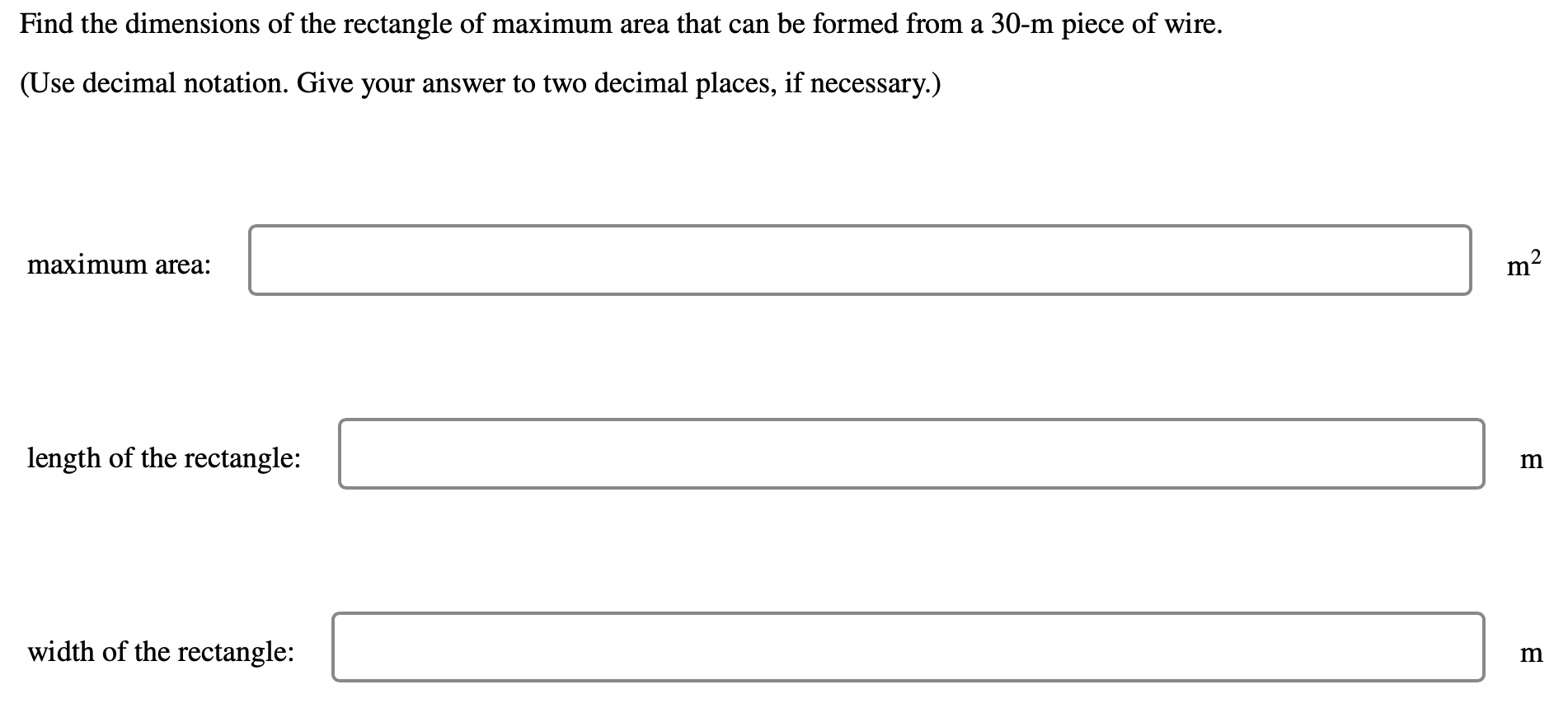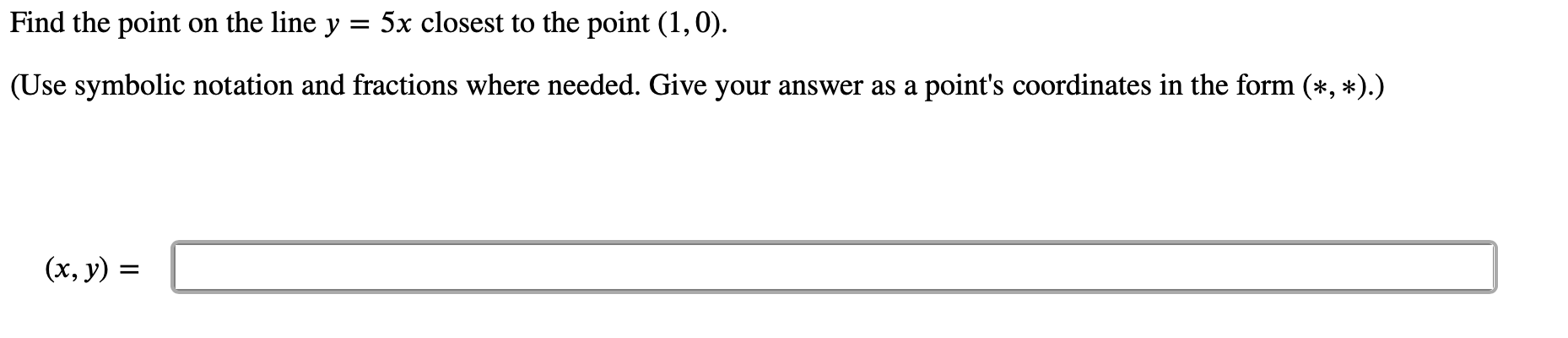# Question Find the dimensions of the rectangle of maximum area that can be formed from a 30-m piece of wire. (Use decimal notation. Give your answer to two decimal places, if necessary.) maximum area: m2 length of the rectangle: m width of the rectangle: m Find the point on the line y = 5x closest to the point (1,0). = (Use symbolic notation and fractions where needed. Give your answer as a point's coordinates in the form (*, *).) (x, y) = =